# Dichotomy

(diff) ← Older revision | Latest revision (diff) | Newer revision → (diff)

The property that for a linear system of ordinary differential equationswith bounded continuous coefficients, there are positive constants,,, andsuch that there exists a decomposition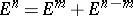for which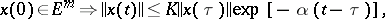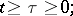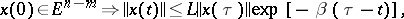(exponential dichotomy; if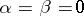, one has ordinary dichotomy). The presence of exponential dichotomy is equivalent to saying that the inhomogeneous systemhas, for any bounded continuous function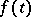,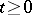, at least one bounded solution on. The theory of dichotomy , transferred to equations in Banach spaces, is also employed in the study of flows and cascades on smooth manifolds .

How to Cite This Entry:
Dichotomy. Encyclopedia of Mathematics. URL: http://encyclopediaofmath.org/index.php?title=Dichotomy&oldid=12757
This article was adapted from an original article by R.A. Prokhorova (originator), which appeared in Encyclopedia of Mathematics - ISBN 1402006098. See original article